XOR Encryption in Python

What You Need

A Kali Linux machine, real or virtual. You could also use OS X, or Windows with Python installed.

Purpose

Encrypt and decrypt files using XOR in Python.

Understanding XOR

Exclusive OR (XOR) is a fundamental mathematical operation used in many encryption algorithms.

XOR operates on one bit at a time, with these results:

0 XOR 0 = 0
0 XOR 1 = 1
1 XOR 0 = 1
1 XOR 1 = 0
For our purposes, we'll use the Python ^ operator, which acts on a whole byte at a time.

Characters are ASCII-encoded, like this:

A is 01000001
B is 01000010
C is 01000011
...
A whole table of ASCII values is here:

Consider A^B:

A is 01000001
B is 01000010
A^B= 00000011
That is character 3, an unprintable end-of-text mark.

However, A^s is printable:

A is 01000001
s is 01110011
A^B= 00110010
The result is the hexadecimal value 0x32, or the numeral 2.

XOR in Python

In Kali Linux, in a Terminal window, execute this command:
nano xor1
In nano, enter the code shown below:
#!/usr/bin/python

import sys

if len(sys.argv) != 4:
print "Usage: ./xor1 infile outfile k"
print "k is a one-character XOR key"
print "For hexadecimal keys, use \$'\\x01'"
exit()

f = open(str(sys.argv), "rb")
g = open(str(sys.argv), "a")
k = ord(sys.argv)

try:
while byte != "":
xbyte = ord(byte) ^ k
g.write(chr(xbyte))
finally:
f.close()

g.close()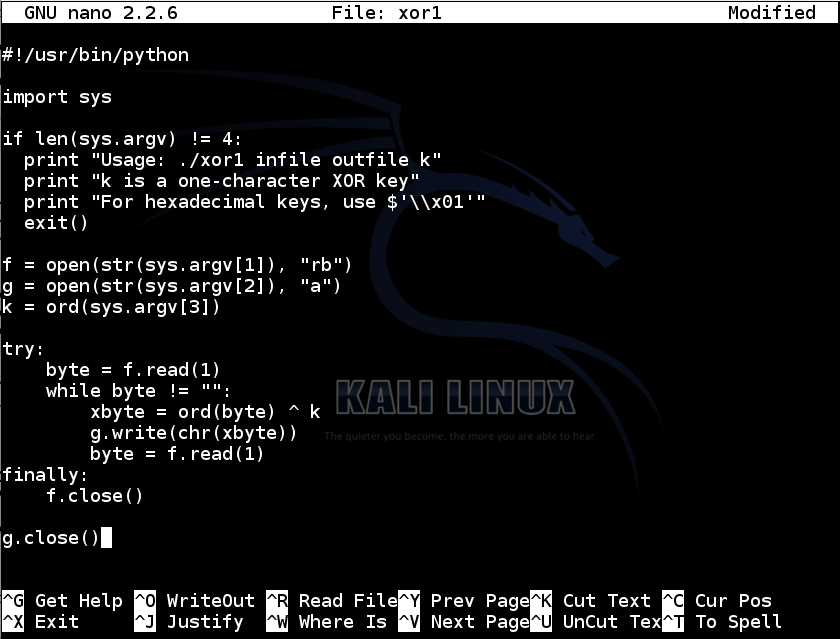Save the file with Ctrl+X, Y, Enter. Next, we need to make the file executable.

In a Terminal window, execute this command:

chmod a+x xor1

Encrypting a Single Character

In a Terminal window, execute this command:
./xor1
You see the help message, explaining how to use the program, as shown below.To create a file named plain1 with the letter A in it, execute these commands :

echo -n A > plain1

cat plain1
The "echo -n" command created a file named plain1 which contains a single letter A, without a carriage return at the end of the file.

The "cat plain1" command printed out the file, which appeared as a single A at the start of the next line, as shown below: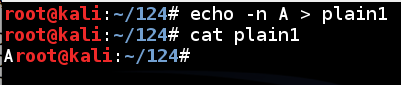To encode the plain1 file with a key of s, execute these commands:

./xor1 plain1 cipher1 s

cat cipher1
The result is 2, as shown below: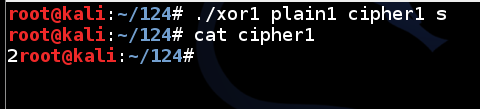Encrypting a Text File

In Kali Linux, in a Terminal window, execute this command:
nano plain2
In nano, enter the code shown below, replacing "YOUR NAME" with your own name:
Normal English text; written by YOUR NAME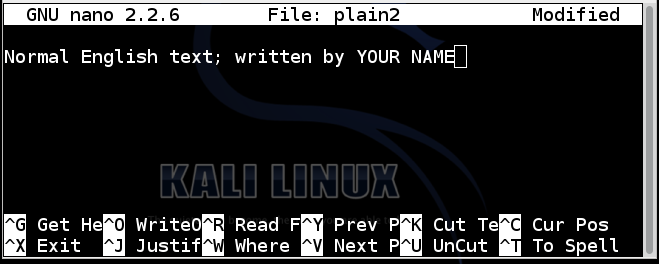Save the file with Ctrl+X, Y, Enter. To encrypt the file using a key of x, execute these commands:

./xor1 plain2 cipher2 x

cat cipher2
The result is strange unreadable characters, as shown below: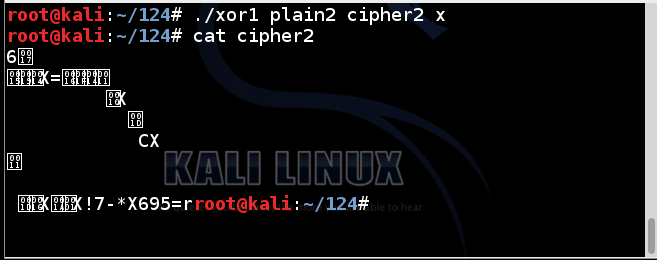Decrypting a Text File

To decrypt a file, simply repeat the XOR operation with the same key. It will reverse itself.

Execute these commands:

./xor1 cipher2 plain2r x

cat plain2r
The file is restored to readable text, as shown below:Last revised: 8-4-16